# Python数据可视化之Plotly使用2020年3月25日07:03:20

2 6618字阅读22分3秒

## Plotly的基本使用

### 准备工作

1. # 绘制动图使用plotly
2. import plotly.express as px

1. gapminder = px.data.gapminder() # 导入需要使用的数据集

• 国家名称
• 所属大洲
• 年份
• 当时的人均寿命
• 当时的人口
• 当时的GDP
• iso_alpha是国家代码(这个我之后我详细说一下)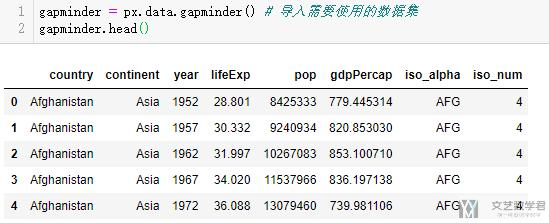### 绘制出某一年的数据-静态图像

• x轴是gdp, y轴是人均寿命
• 散点图的颜色代表着国家所属的大洲
• 每个点的颜色代表着这个国家的人口
1. gapminder2002 = gapminder.query('year == 2002')
2. px.scatter(gapminder2002, x='gdpPercap', y='lifeExp', color='continent', size='pop', size_max=60)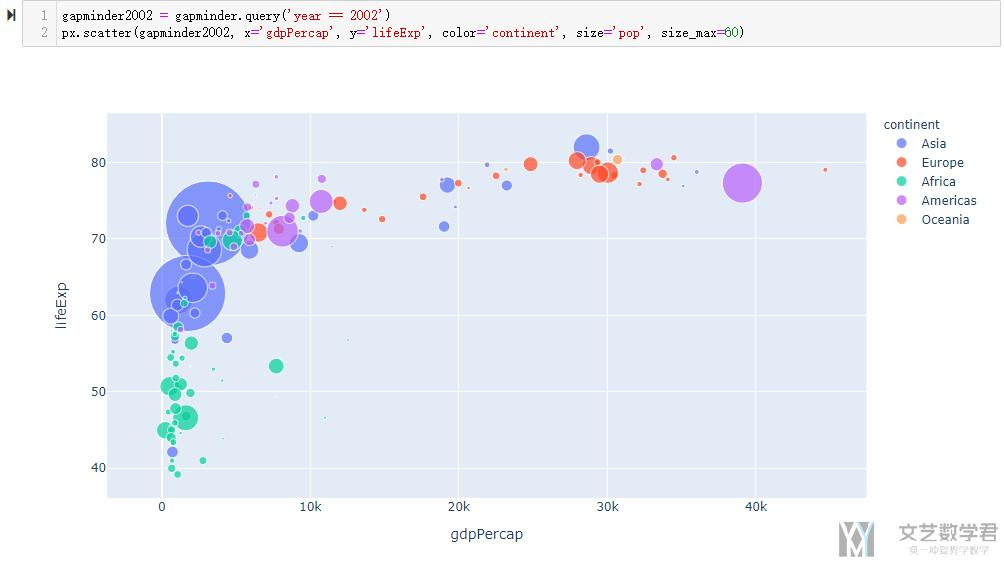### 静态图像的互动

1. gapminder2002 = gapminder.query('year == 2002')
2. px.scatter(gapminder2002, x='gdpPercap', y='lifeExp',
3.            color='continent', size='pop', size_max=60,
4.            hover_name="country", # 悬停显示的内容
5.            hover_data=["year","continent","gdpPercap","lifeExp"],
6.           title="Mathpretty")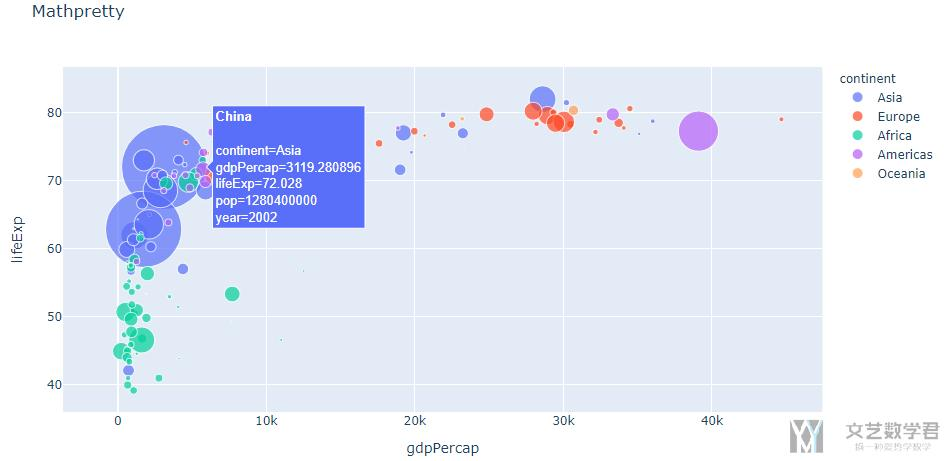### 子图的绘制

1. gapminder2002 = gapminder.query('year == 2002')
2. px.scatter(gapminder2002, x='gdpPercap', y='lifeExp',
3.            color='continent', size='pop', size_max=60,
4.            hover_name="country", # 悬停显示的内容
5.            hover_data=["year","continent","gdpPercap","lifeExp"],
6.            facet_col='continent',
7.           title="Mathpretty")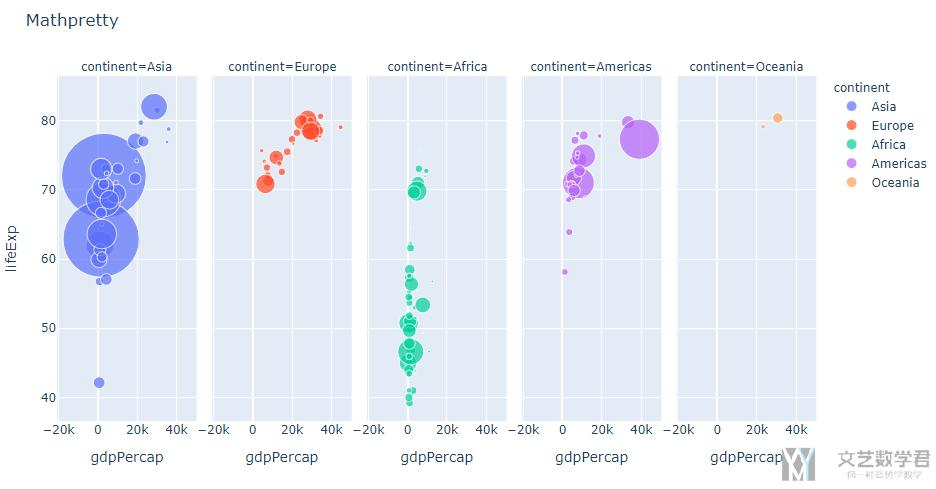### 动态图的绘制

• animation_frame: 这个就可以理解为我们根据什么动, 这里就是根据时间
• animation_group: 这个可以理解为我们的主体是什么, 这里是国家
• range_x, range_y: 这个没什么好解释的, 就是x轴和y轴的范围
• labels: 有的时候我们的label不是那么好懂, 可以使用这个来改变labels的显示的名称.
1. px.scatter(gapminder, x='gdpPercap', y='lifeExp',
2.            color='continent', size='pop', size_max=60,
3.            animation_frame='year', animation_group='country',
4.           range_y = [30,100], range_x = [-5000,55000],
5.           labels = {'gdpPercap':'GDP', 'lifeExp':'Life Expectancy'})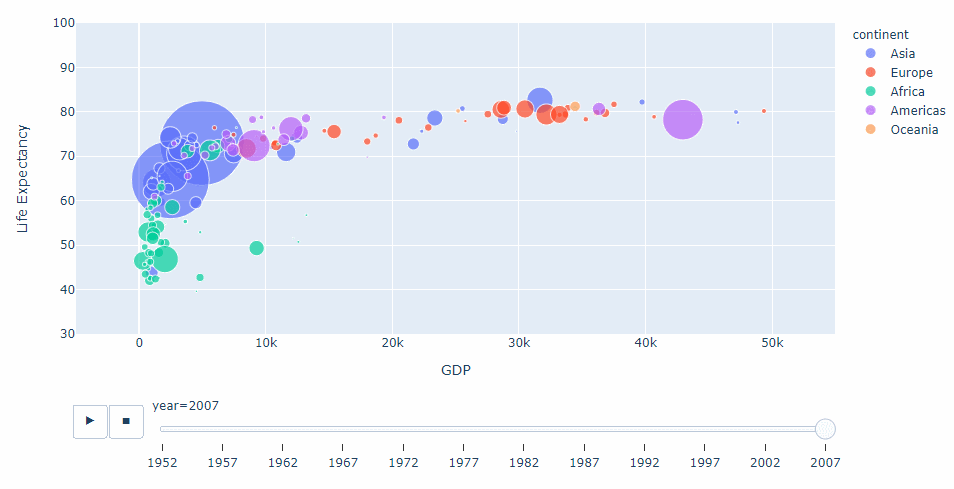## Plotly绘制关于地理的图形

### 区域着色

1. fig = px.choropleth(gapminder,
2.                     locations = 'iso_alpha',
3.                     color='lifeExp',
4.                     animation_frame="year",
5.                     color_continuous_scale=px.colors.diverging.RdBu,
6.                     projection = 'natural earth')
7. fig.update_layout(title='World GDP v.s. LifeExp',
8.                   font=dict(family="Courier New, monospace",size=18,color="#7f7f7f")
9.                  )
10. fig.show()

• locations: 这个是接受国家每个国家的ISO3代码的, 也就是China就是CHN, 我们下面会解释如何进行转换.
• projection: 这个是可以选择地图的类型, 我觉得世界地图还是下面这种比较好看.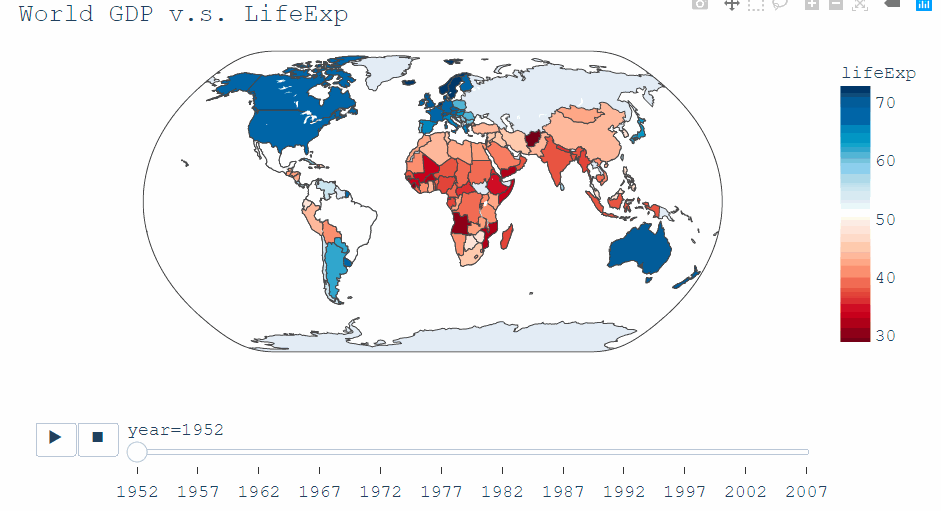### 关于气泡地图

1. fig = px.scatter_geo(gapminder2002, locations="iso_alpha", color="continent",
2.                      hover_name="country", size="pop",
3.                      size_max = 30,
4.                      projection="natural earth")
5. fig.show()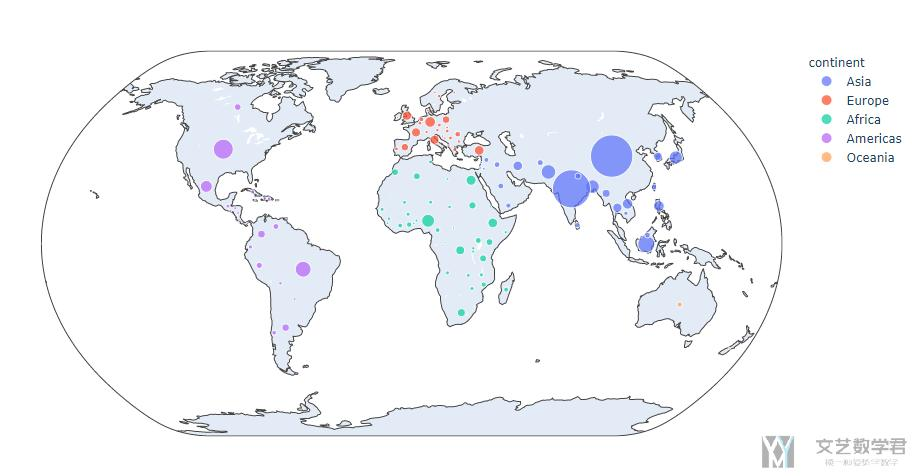1. fig = px.scatter_geo(gapminder, locations="iso_alpha", color="continent",
2.                      hover_name="country", size="pop",
3.                      size_max = 30,
4.                      animation_frame='year', animation_group='country',
5.                      projection="natural earth")
6. fig.show()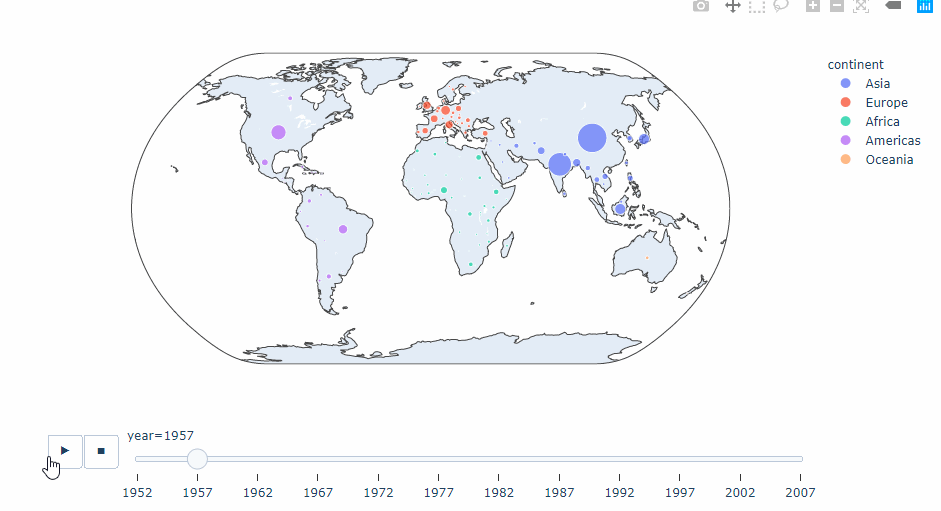## Plotly高级绘图

### 玫瑰图(Wind Rose)

wind rose也称作polar bar chart, 下面数据集是每个方向的风力, 我们将其绘制出玫瑰图来.

1. df = px.data.wind()
2. fig = px.bar_polar(df, r="frequency", theta="direction",
3.                    color="strength", template="plotly_dark",
4.                    color_discrete_sequence= px.colors.sequential.Plasma_r)
5. fig.show()

• r是代表半径
• theta代表角度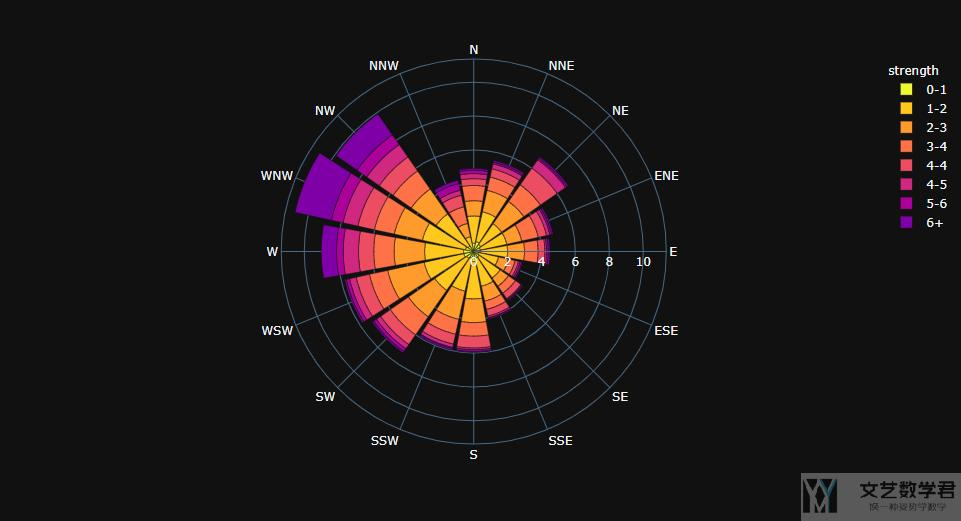1. import plotly.graph_objects as go
2. fig = go.Figure()
4.     r=[77.5, 72.5, 70.0, 45.0, 22.5],
5.     name='A',
6.     marker_color='rgb(106,81,163)'
7. ))
9.     r=[57.5, 50.0, 45.0, 35.0, 20.0],
10.     name='B',
11.     marker_color='rgb(158,154,200)'
12. ))
14.     r=[40.0, 30.0, 30.0, 35.0, 7.5],
15.     name='C',
16.     marker_color='rgb(203,201,226)'
17. ))
18. fig.update_traces(text=['A', 'B', 'C', 'D', 'E'])
19. fig.update_layout(
20.     title='A Test',
21.     #font_size=16,
22.     legend_font_size=16,
24.     polar_angularaxis_rotation=90,
25. )
26. fig.show()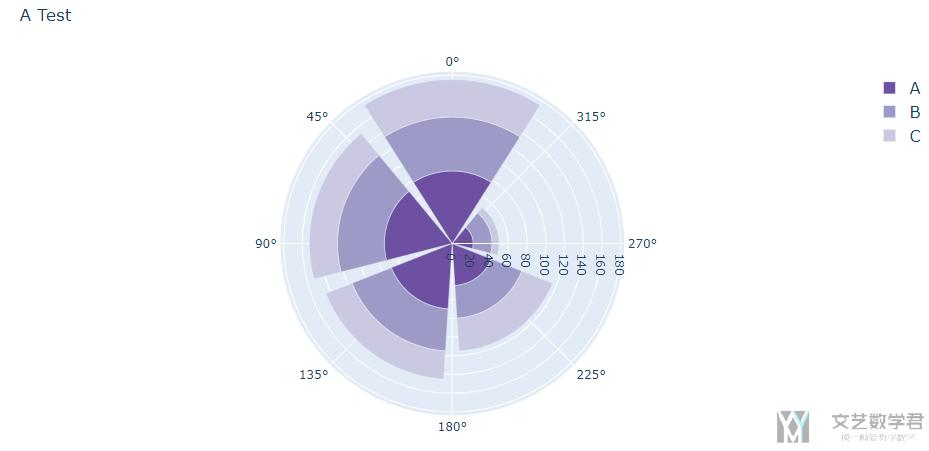### Sunburst图的绘制

1. df = px.data.gapminder().query("year == 2007")
2. fig = px.sunburst(df, path=['continent', 'country'], values='pop',
3.                   color='lifeExp', hover_data=['iso_alpha'],
4.                   color_continuous_scale='RdBu',
5.                   color_continuous_midpoint=np.average(df['lifeExp'], weights=df['pop']))
6. fig.show()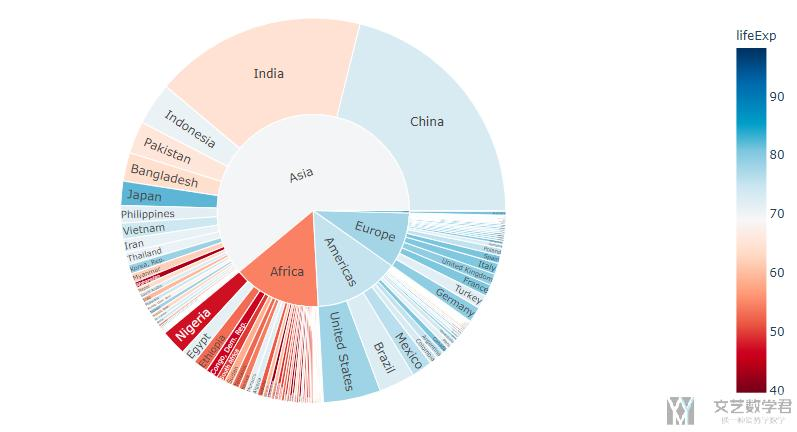1. data = dict(
2.     character=["Eve", "Cain", "Seth", "Enos", "Noam", "Abel", "Awan", "Enoch", "Azura"],
3.     parent=["", "Eve", "Eve", "Seth", "Seth", "Eve", "Eve", "Awan", "Eve" ],
4.     value=[42, 14, 12, 10, 2, 6, 6, 4, 4]) # 这里的value对应上面的character
5. fig =px.sunburst(
6.     data,
7.     names='character',
8.     parents='parent',
9.     values='value',
10.     branchvalues='total',
11.     color = 'value'
12. )
13. fig.update_layout(showlegend=True)
14. fig.show()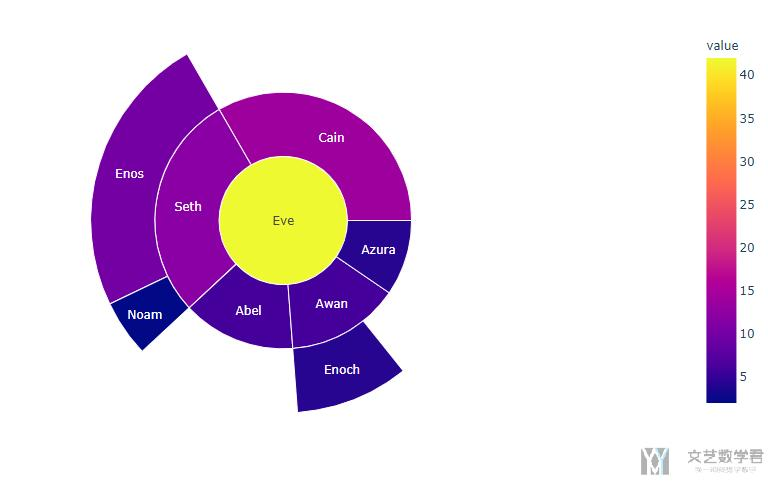## Plotly其他注意事项

### 关于Plotly配色问题

Plotly配色是给了三套配色(大类), 分别是:

• 层次渐变, Sequential Color scales
• 强烈的对比渐变, Diverging Color scales
• 循环渐变, Cyclical Color scales

### 绘制Map类型时国家代号转换

• 微信公众号
• 关注微信公众号
•• QQ群
• 我们的QQ群号
•• 本文由 发表于 2020年3月25日07:03:20
• 转载请务必保留本文链接：https://mathpretty.com/11919.html